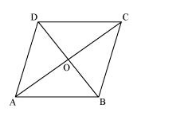# Three vertices of a parallelogram ABCD are A (3, –1, 2), B (1, 2, –4) andC (–1, 1, 2). Find the coordinates of the fourth vertex.

Question:

Three vertices of a parallelogram ABCD are A (3, –1, 2), B (1, 2, –4) andC (–1, 1, 2). Find the coordinates of the fourth vertex.

Solution:

The three vertices of a parallelogram ABCD are given as A (3, –1, 2), B (1, 2, –4), and C (–1, 1, 2). Let the coordinates of the fourth vertex be D (xyz).We know that the diagonals of a parallelogram bisect each other.

Therefore, in parallelogram ABCD, AC and BD bisect each other.

$\therefore$ Mid-point of $A C=$ Mid-point of $B D$

$\Rightarrow\left(\frac{3-1}{2}, \frac{-1+1}{2}, \frac{2+2}{2}\right)=\left(\frac{x+1}{2}, \frac{y+2}{2}, \frac{z-4}{2}\right)$'

$\Rightarrow(1,0,2)=\left(\frac{x+1}{2}, \frac{y+2}{2}, \frac{z-4}{2}\right)$

$\Rightarrow \frac{x+1}{2}=1, \frac{y+2}{2}=0$, and $\frac{z-4}{2}=2$

$\Rightarrow x=1, y=-2$, and $z=8$

Thus, the coordinates of the fourth vertex are (1, –2, 8).